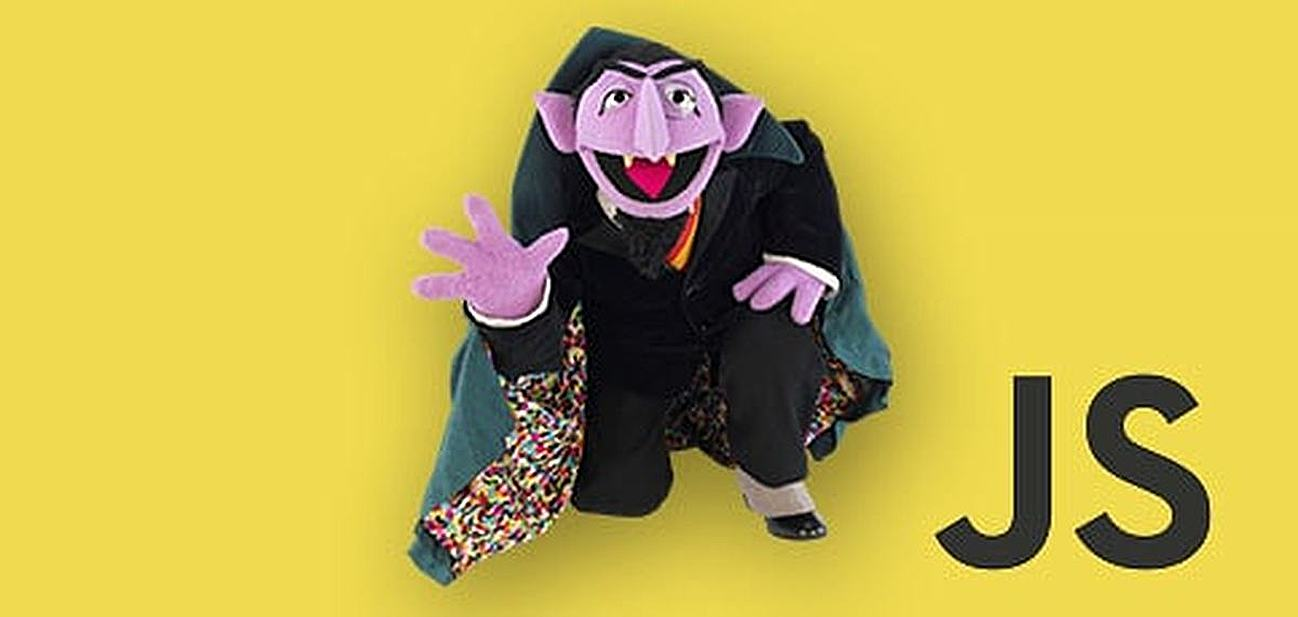# JavaScript: is numberDeciding whether something is a number in JavaScript should be a straightforward type check (i.e., using the isNaN() function), but, as it turns out, it is a bit more tricky.

## How to Determine Whether or Not Something is a Number in JavaScript

This is due to JavaScript’s forced conversion-to-number behavior. The often used methods, isNaN() and isFinite() can have confusing behavior and return incorrect answers.

Thankfully, ECMAScript 6 (JS language specification) was released with the intention of fixing these issues with Number.isNaN() and Number.isFinite(), along with making many other language clarifications and improvements.

### Use the isNumeric() Function

Until that JS language version is the standard and used in browsers, we will need to be careful when checking if a value is a number.

The jQuery project has a tiny isNumeric() function implementation that fixes this odd JS behavior. There are some other implementations, but many of those fail in some of the JS type-casting-gotcha corner cases.

The isNumeric() function below is usable in your own code, even without jQuery.

```var isNumeric = function(obj){
return !Array.isArray( obj ) && (obj - parseFloat( obj ) + 1) >= 0;
}```

### isNumeric() Examples

Below are some examples of using isNumeric() with its boolean output:

```isNumeric(10); // true
isNumeric(“0.75”); // true
isNumeric(“123e–2”); // true
isNumeric(–0xFFF); // true

isNumeric(Infinity); // false , use isFinite()
isNumeric(NaN); // false , use isNaN()
isNumeric(); // false
isNumeric(true); // false
isNumeric(“foo”); // false
isNumeric(null); // false
isNumeric(new Date()); // false```